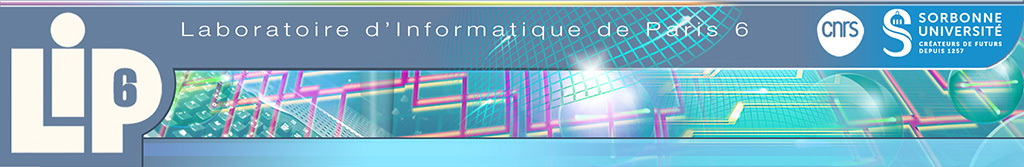Extending Constructive Solid Geometry to Projections and Parametric Objects

04/04/2014
Palestrante(s) : George Tzoumas (CNRS UMR 5158, LE2I, University of Burgundy)
Constructive Solid Geometry (CSG) is a widely used representation scheme in Computer Aided Design and Manufacturing (CAD/CAM). Solids are represented as Boolean constructions via regularized set operators. CSG representations are essentially binary expression trees with non-terminal nodes representing operators and terminal nodes typically representing primitive solids (like spheres). We extend traditional CSG trees to support the projection operator. Existing algorithms in the literature compute various topological properties of CSG sets. Our extension readily allows these algorithms to work on a greater variety of sets, in particular parametric sets, which are extensively used in CAD/CAM systems. A geometric primitive may be defined in terms of a characteristic function, which can be seen as the zero-set of a corresponding system along with inequality constraints. To handle projections, we exploit the Disjunctive Normal Form, since projection distributes over union. To handle intersections, we transform them into disjoint unions. Each point in the projected space is mapped to a contributing primitive in the original space. This way we are able to perform gradient computations on the boundary of the projected set through equivalent gradient computations in the original space. By traversing the final expression tree, we are able to automatically generate a set of equations and inequalities that express either the geometric solid or the conditions to be tested for computing various topological properties, such as homotopy equivalence.
Web page
Elias.Tsigaridas (at) nulllip6.fr